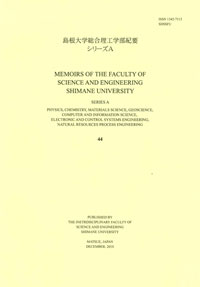# Memoirs of the Graduate School of Science and Engineering Shimane University. Series A

ISSN:13427113Memoirs of the Graduate School of Science and Engineering Shimane University. Series A 33
1999-12-24 発行

# 静的曲げ試験における改造したTimoshenkoの曲げの式の適用範囲

Applicable Range of the Modified Timoshenko's Bending Equation on the Staic Bending Tests
Description
This paper describes the examination about the proper range of depth/span ratio which gives the Young's modulus and shear mqdulus in the three-point static bending test, and obtained the following results.
(1) The applicable depth/span ratio range obtained from our proposal is wider than that given by Timoshenko's theory. However, the Young's modulus cannot be given when the depth/span ratio is large, whereas the shear modulus cannot be derived when the depth/span ratio is small. Hence, the range of depth/span ratio should be wide in obtaining the both values of Young's modulus and shear modulus by our proposal properly.
(2) The accuracy of shear modulus obtained from our proposal is dependent on the value of the coefficient used for the modification of original Timoshenko's bending theory. For obtaining the proper shear modulus, this coefficient should be determined carefully by considering the various factors including the mechanical properties of the specimen themselves and the experimental conditions such as the loading nose used and the measured point of deflection.
(3) It was thought that the shear modulus can be derived from the bending test of one specimen as well as the Young's modulus. Nevertheless, the feasibility is restricted by the depth/span ratio.
NCID
AA11157087